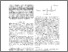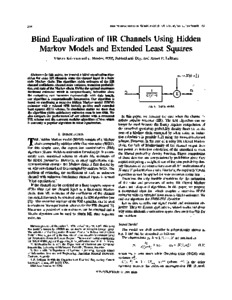# Blind Equalization of IIR Channels Using Hidden Markov Models and Extended Least Squares

Krishnamurthy, Vikram and Dey, Subhrakanti and LeBlanc, James P (1995) Blind Equalization of IIR Channels Using Hidden Markov Models and Extended Least Squares. IEEE Transactions on Signal Processing, 43 (12). pp. 2994-3006. ISSN 1053-587XPreviewmore...Add this article to your Mendeley library

## Abstract

In this paper, we present a blind equalization algorithm for noisy IIR channels when the channel input is a finite state Markov chain. The algorithm yields estimates of the IIR channel coefficients, channel noise variance, transition probabilities, and state of the Markov chain. Unlike the optimal maximum likelihood estimator which is computationally infeasible since the computing cost increases exponentially with data length, our algorithm is computationally inexpensive. Our algorithm is based on combining a recursive hidden Markov model (HMM) estimator with a relaxed SPR (strictly positive real) extended least squares (ELS) scheme. In simulation studies we show that the algorithm yields satisfactory estimates even in low SNR. We also compare the performance of our scheme with a truncated FIR scheme and the constant modulus algorithm (CMA) which is currently a popular algorithm in blind equalization.

Item Type: Article Blind Equalization; IIR Channels; Hidden Markov; Models; Extended Least Squares; Faculty of Science and Engineering > Electronic Engineering 12735 https://doi.org/10.1109/78.476443 Subhrakanti Dey 14 Apr 2020 10:37 IEEE Transactions on Signal Processing IEEE YesItem control page